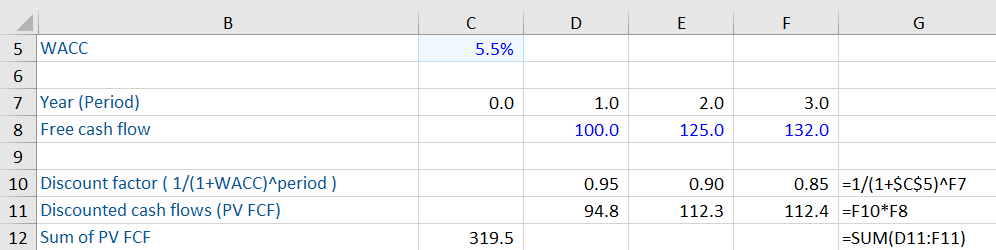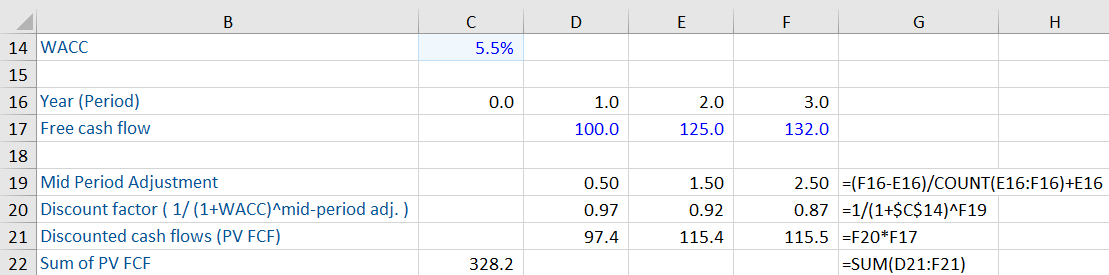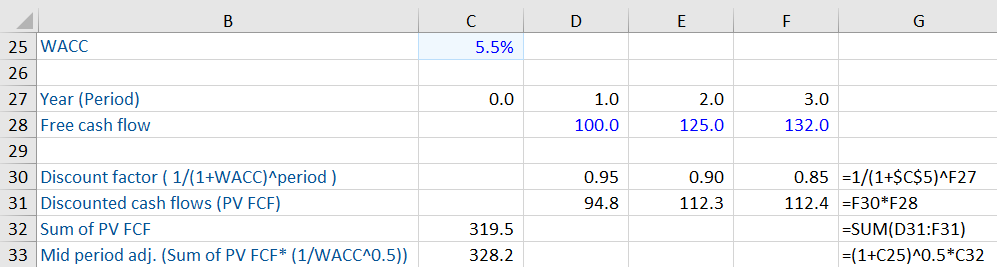## What is “Mid Period”?

Mid period refers to the halfway point in a cash flow forecast period, used as a proxy for when the cash flows will be received on average.  When valuing cash flows using the discounted cash flow method, we often use the mid period to reflect that cash flows occur throughout the period, and not just at the end.  The traditional valuation method discounts cash flows back from the end of a period of time; however, this can undervalue an investment as cash flows at the end of the year are farther away from today from the time value of money perspective.

## Key Learning Points

• Mid Period is the halfway point between the beginning and end of a forecast period
• It is used to assume that cash flows are most likely to fall on average, in the middle of a discounting period as opposed to at the beginning or end of a period
• A Mid Period adjustment will return a value greater than a full year adjustment since the cash flow is assumed to occur a half period closer in time than the end of period
• The Mid Period adjustment can be applied to any period of time being used in a forecast but it must be used consistently with any terminal value calculation that is based on cash flows

## Calculating the Mid Period

The mid period is represented as the halfway point between two periods of time.  In a five year cash flow forecast, the periods would be 0 (starting point),1,2,3,4 and 5.  The mid period of each period would be the average of the beginning period and the end period or 0.5, 1.5, 2.5, 3.5, 4.5.

## Mid Year Example Calculation

The mid period will be used in calculating the discount factor for each period.  In the first example we see that the mid period adjustment has not been used.  The cash flows are being discounted from the end of each period.In this second example, we see that the mid period adjustment has been calculated (row 19) and that has resulted in a lower discount factor (row 20) and, therefore, greater sum of the present value of free cash flows (C22).In the last example, we see that the mid period adjustment can be applied to the sum of the present value of the free cash flows in the first example and return the same result as the second example.  This is useful in avoiding the step of adjusting each period’s discount factor.## Applications and Other Considerations

As discussed, the mid period adjustment is commonly used in a discounted cash flow calculation.  When applied to the calculation of the discount factor, it results in a larger factor that returns a greater value than discounting for the whole period.   If the mid period adjustment is used to discount the free cash flows in the forecast period, it must also be used to discount the terminal value to present value when using the perpetual cash flow (Gordon Growth) methodology.  This is because we are assuming the perpetual cash flows also fall on average at mid period.

## Conclusion

Mid period adjustments bring greater accuracy to a discounted cash flow valuation by assuming cash flows fall on average at the middle of the period instead of the end.  This is because the total cash flows from a given period do not occur at a single point in time, but rather fall throughout the period.